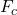Question

A charged particle is placed in an external magnetic field and it is moving in a circular path of radius 26m.The magnetic force acting on the particle is 16N.What is the kinetic energy of the particle

1.thienan

208 Joules

Explanation:

The radius of the circular path the charge moves, r = 26 m

The magnetic force acting on the charge particle, F = 16 N

Centripetal force,= m·v²/r

Kinetic energy, K.E. = (1/2)·m·v²

Where;

m = The mass of the charged particle

v = The velocity of the charged particle

r = The radius of the path of the charged particle

Whereby the magnetic force acting on the charge particle = The centripetal force, we have;

F == m·v²/r = 16 N

(1/2) × r ×= (1/2) × r × m·v²/r = (1/2)·m·v² = K.E.

∴ (1/2) × r ×= (1/2) × 26 m × 16 N =  = (1/2)·m·v² = K.E.

∴ 208 Joules = K.E.

The kinetic energy of an particle moving in the circular path, K.E. = 208 Joules.